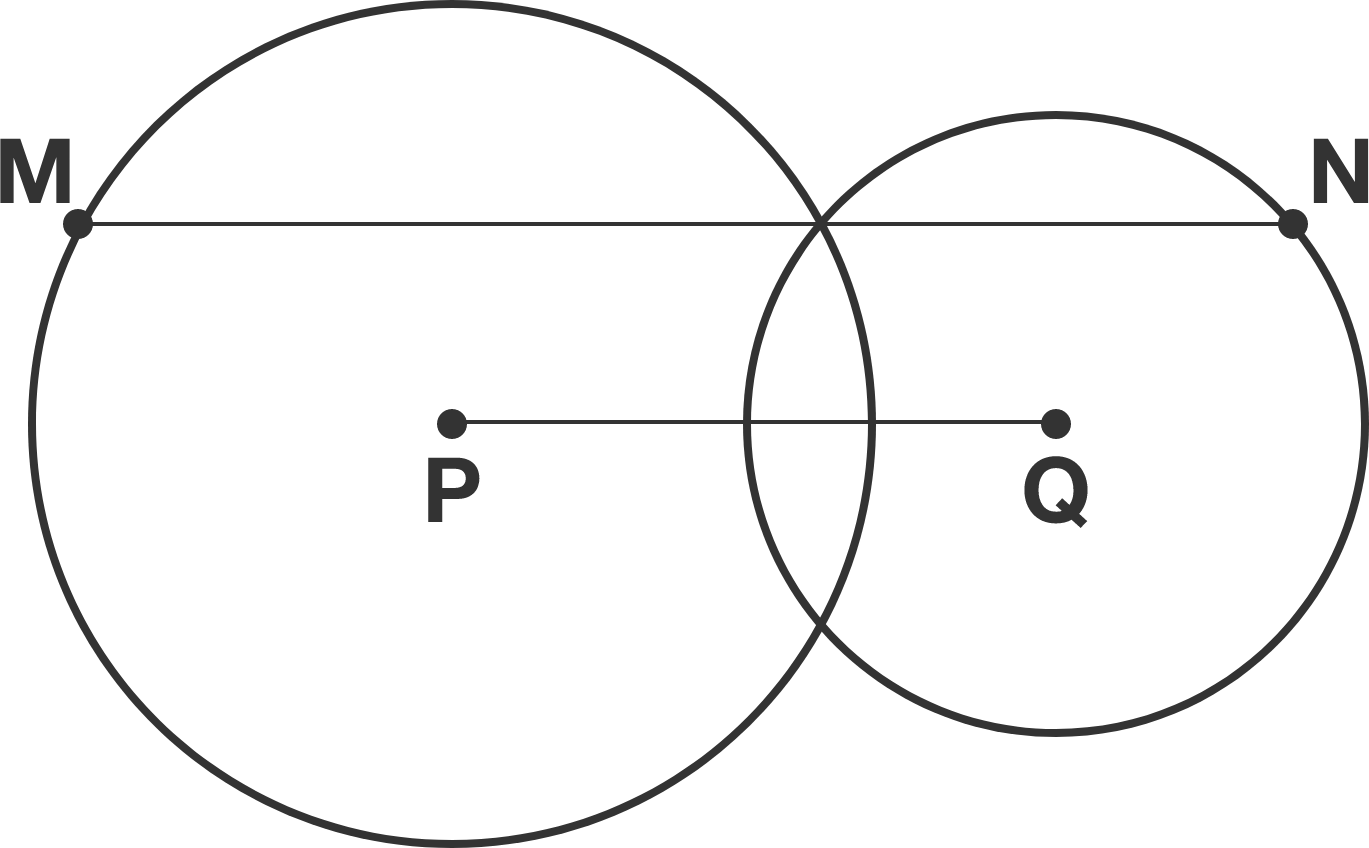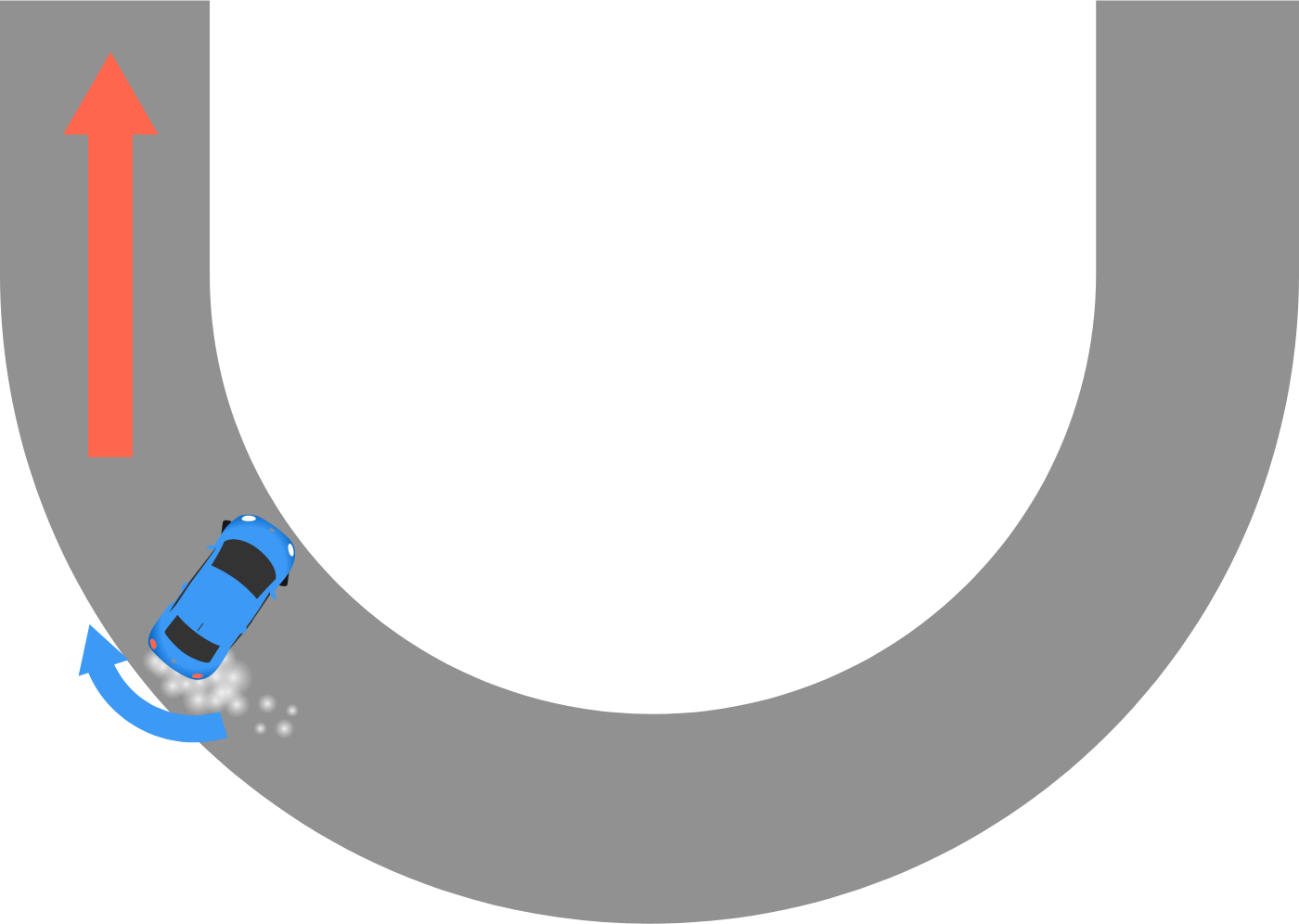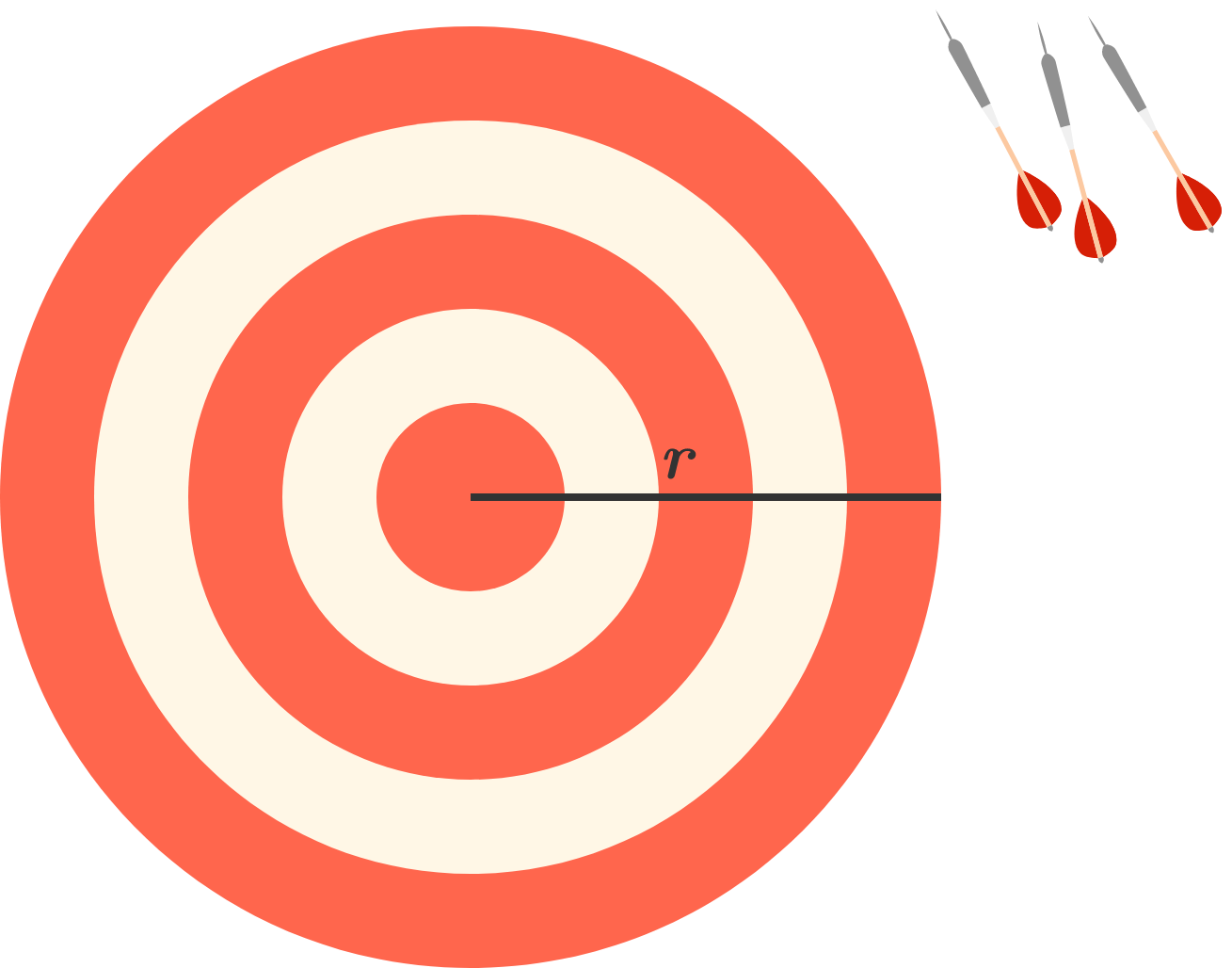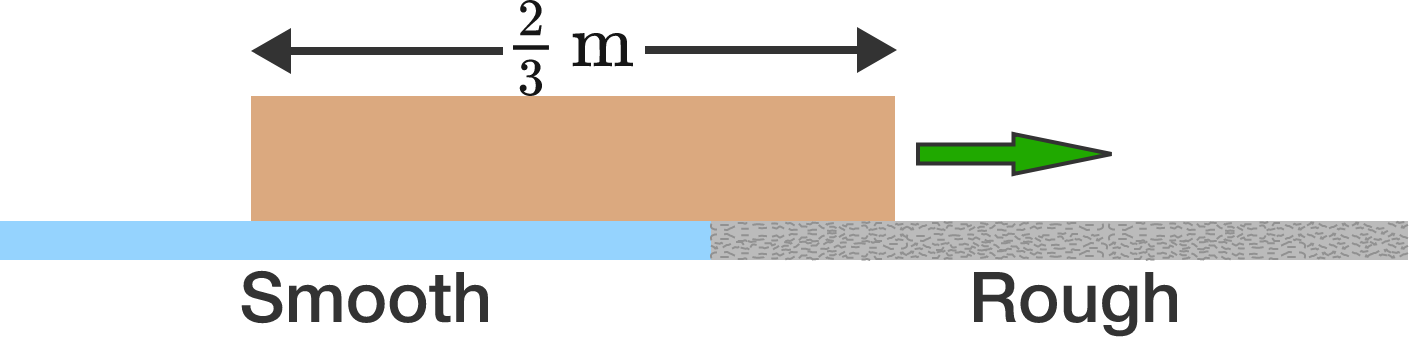# Problems of the Week

Contribute a problem

# 2017-10-30 Intermediate

Two circles with respective centers $P$ and $Q$ intersect with each other, as shown below. Line segment $MN$ is parallel to $PQ$ and goes through one of the intersection points.

If $PQ=3,$ what is $MN?$"Drifting" is a method of turning in a car race. After starting the turn, the driver sharply turns the steering wheel in the opposite direction of the turn. The rear wheels, powered by the engine, continue to spin fast while the car's rear slides out from the center of the turn.

In the figure below, the driver is drifting out of a right turn, and the steering wheel is turned to the left. What should the driver do next to finish the turn and go straight?1. Turn the steering wheel to the right. That will line up the front wheels with the rear wheels and make the car go straight.
2. Turn the steering wheel more to the left. That will create a torque on the car that stops the car's rotation around the vertical axis.
3. Keep the steering wheel steady and step on the gas more strongly to get a larger forward force from the rear wheels.
4. Keep the steering and the gas unchanged and hope for the best.

Amy is a cashier at a convenience store and has run out of all dollar bills. But she has plenty of coins--pennies (1 cent), nickels (5 cents), dimes (10 cents), quarters (25 cents)--in the cash drawer, and is well trained for an emergency situation like this: give the change with the fewest possible coins!

She just gave a change with 20 coins. What is the minimum possible value of the change, in cents?

Clarification: For example, if you think the change she just gave her customer is 2 dollars and 37 cents, write 237.

You need to be connected to run code

Bonus Question: What's the general formula when the number of coins is not 20 but some integer $n\ge 6?$

You are given a dartboard of radius $r$ and an infinite number of darts. When you throw a dart at the dartboard, it always strikes the dartboard, but you don't necessarily control where it strikes.

You are about to throw $n$ darts. What is the minimum value of $n$ such that there must be a pair of darts that are closer than $r$ to each other?Note: The point of each dart is one-dimensional. Darts can be thrown onto the edge of the dartboard.A rectangular box of length $\frac{2}{3} \text{m}$ is initially traveling at $2 \text{ m/s},$ with its entire length over the smooth (perfectly frictionless) blue surface (shown above). The box gradually moves onto a rough surface (gray) and stops the instant that its entire length is positioned within the rough region.

Determine the coefficient of kinetic friction.


Details and Assumptions:

• $g = 10\text{ m/s}^2.$
• The pressure at the bottom of the box is always uniform over its area.
×